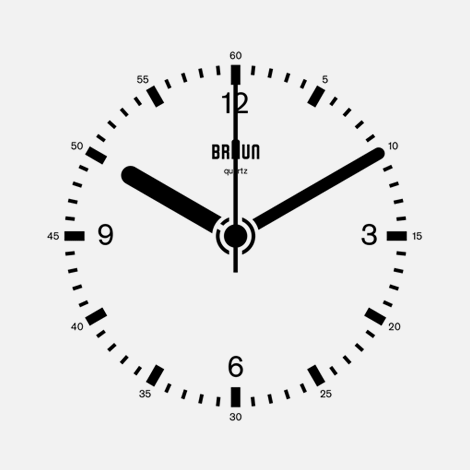# Clock Problems

## Clock Problems Shortcut Tricks

A clock or a watch is an instrument which shows time at a moment. A clock has two hands, smaller hand is called short hand or hour hand. While larger hand is called long hand or minute hand.### Importation Point for Clock Problem

• A clock has two hands, smaller hand is called short hand or hour hand. While larger hand is called long hand or minute hand.
• Both hands of clock coincides in one hour
• In each 60 minutes, the minute hand gains 55 minutes on the hour hand
• When both the hands are at right angle, they are 15 minutes space apart
• Both hands are in straight line when they are opposite to each other or coincident to each other.
• Angle traced by hour hand in 12 hours = 360°
• Angle traced by minute hand in 60 minutes are 360°
• When both hour and minute hands are at right angle they are 15 minute apace apart

• (a) 60°
• (b) 45°
• (c) 75°
• (d) 90°

## Solution

```At 3:30 , the minute hand is at number 6.
At 3:00 the hour hand was at number 3.
Now it has moved 15 degree.
Hence the angle between the two is ( 90 -15) = 75 degree.
```